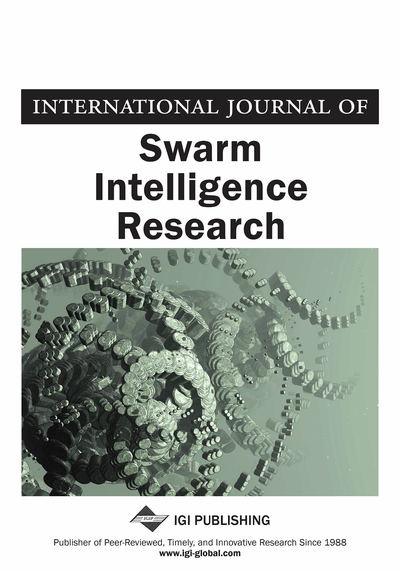# Application of Water Cycle Algorithm to Stochastic Fractional Programming Problems

Prachi Agrawal (National Institute of Technology, Hamirpur, India), Talari Ganesh (National Institute of Technology, Hamirpur, India) and Ali Wagdy Mohamed (Cairo University, Egypt)
DOI: 10.4018/IJSIR.2022010101
Available
\$37.50
No Current Special Offers

## Abstract

This paper presents an application of Water Cycle algorithm (WCA) in solving stochastic programming problems. In particular, Linear stochastic fractional programming problems are considered which are solved by WCA and solutions are compared with Particle Swarm Optimization, Differential Evolution, and Whale Optimization Algorithm and the results from literature. The constraints are handled by converting constrained optimization problem into an unconstrained optimization problem using Augmented Lagrangian Method. Further, a fractional stochastic transportation problem is examined as an application of the stochastic fractional programming problem. In terms of efficiency of algorithms and the ability to find optimal solutions, WCA gives highly significant results in comparison with the other metaheuristic algorithms and the quoted results in the literature which demonstrates that WCA algorithm has 100% convergence in all the problems. Moreover, non-parametric hypothesis tests are performed and which indicates that WCA presents better results as compare to the other algorithms.
Article Preview
Top

## 1. Introduction

Stochastic programming deals with the condition where the parameters of the problem are uncertain or random e.g., future demands of goods depend on the market conditions; the transportation cost depends on the uncertain price of fuel, and crop yields typically depend on the weather conditions (Birge and Louveaux 2011). The uncertain parameters of the problem are considered as random variables that follows probability distribution (Rao 2019). To solve the stochastic programming problem, the idea is to convert a probabilistic problem into its equivalent deterministic form, and then the solutions are obtained by classical/ numerical techniques. Stochastic programming has been widely used in various areas of science and engineering. It has been applied to different real-time problems such as transportation (Yang and Feng 2007), finance (Carino et al. 1994), electrical generation capacity planning (Murphy et al. 1982), portfolio optimization (Abdelaziz et al. 2007), medical field (Ben and Masmoudi 2012, Punnakitikashem 2008), supply chain management (Fisher et al. 1997), social network (Wu and Küçükyavuz 2018), Risk Criterion (Riis and Schultz 2003). In this paper, stochastic fractional programming (SFP) problem is considered, which optimizes the ratio of two functions with some constraints. At least one of the parameters must be uncertain (or random) in nature, or some of the constraints can be in deterministic form. SFP problems have been applied to the business sector in different scenarios (e.g., cost v/s time, cost v/s profit, cost v/s volume). There are several classical and numerical techniques to solve the SFP problems (Charles and Dutta 2001, 2004, 2005, Charles et al. 2001). Mohamed (2017) proposed enhance adaptive differential evolution algorithm and Hadi et al. (2019) proposed LSHADE-SPA to solve high dimensional and complex optimization problems. In the last three decades, metaheuristic algorithms developed to solve the complex-computational, high-dimensional, non-differentiability, non-convexity problems. Basically, metaheuristic algorithms depend on the natural evolution and the algorithms are started with a randomly generated population. The most popular algorithms are Genetic Algorithm (GA) (Holland 1992), Particle Swarm Optimization (PSO) (Eberhart and Kennedy 1995), Differential Evolution (DE) (Das and Suganthan 2010), Ant Colony Optimization (ACO) (Dorigo and Birattari 2010), Teaching Learning Based Optimization (TLBO) (Rao 2016) etc. There are other several metaheuristic algorithms which are used to solve real-world applications such as energy efficiency in the IoT networks (Iwendi et al. 2020), early detection of diabetic retinopathy (Gadekallu et al. 2020), XGBoost classification model for intrusion detection (Bhattacharya, 2020), global optimization problems (Wu et al., 2020) etc. To solve the stochastic programming, Thangaraj et al. (2011) and Mohamed (2017) used DE algorithm and found the optimal solution.

## Complete Article List

Search this Journal:
Reset
Open Access Articles
Volume 13: 4 Issues (2022): 3 Released, 1 Forthcoming
Volume 12: 4 Issues (2021)
Volume 11: 4 Issues (2020)
Volume 10: 4 Issues (2019)
Volume 9: 4 Issues (2018)
Volume 8: 4 Issues (2017)
Volume 7: 4 Issues (2016)
Volume 6: 4 Issues (2015)
Volume 5: 4 Issues (2014)
Volume 4: 4 Issues (2013)
Volume 3: 4 Issues (2012)
Volume 2: 4 Issues (2011)
Volume 1: 4 Issues (2010)
View Complete Journal Contents Listing